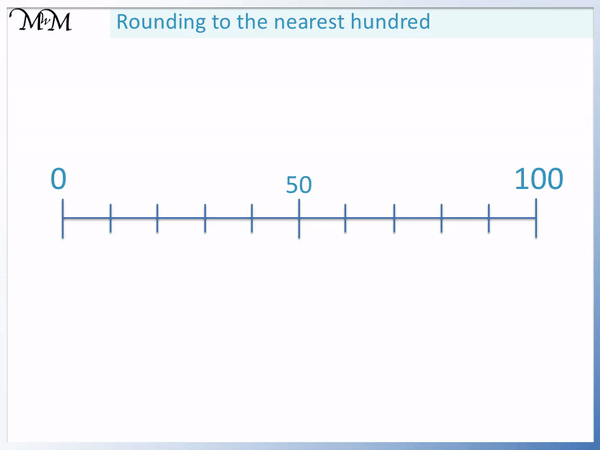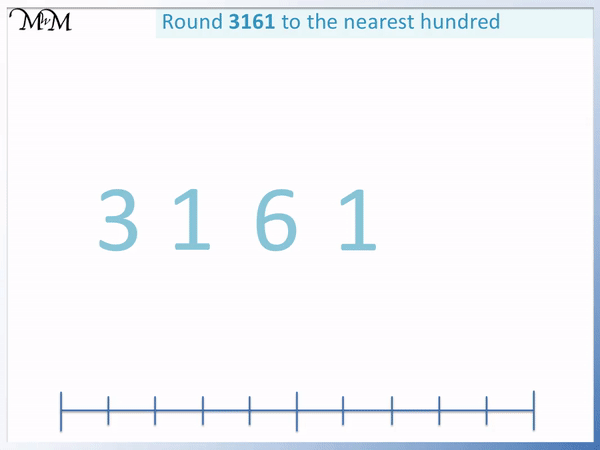# Rounding to the Nearest Hundred

Rounding to the Nearest Hundred#### How to Round to the Nearest Hundred

Look at the digit in the tens column.

If it is 4 or less, round down. If it is 5 or more, round up.To round up, increase the hundreds digit by one and set the following digits to zero.

To round down, keep the hundreds digit the same and set the following digits to zero.

• Rounding to the nearest 100 means to write the multiple of 100 that is nearest to the number.
• Either the hundreds digit will remain the same or it will increase by one.
• 379 is in between 300 and 400, so the options are to round down to 300 or round up to 400.
• To decide whether to round up or down, look at the digit in the tens column.
• If the digit is 4 or less, round down.
• If the digit is 5 or more, round up.
• This is because 350 is halfway between 300 and 400.
• 379 has a 7 as its tens digit, which is ‘5 or more’.
• This means that 379 rounds up to 400.
• This means that 379 is nearer to 400 than 300.
• Always look at the tens digit to decide how to round a number to the nearest hundred.• 849 has an 8 as its hundreds digit so we can choose to round down to 800 or round up to 900.
• We look at the digit in the tens column, which is 4.
• This digit is ‘4 or less’ and so we round down.
• 849 rounds down to 800.
• This means that 849 is nearer to 800 than 900.
• We can see that it is just before 850, which is the halfway point between 800 and 900.#### Rounding to the Nearest Hundred Game

Click on the link below to practise rounding to the nearest hundred with this interactive game.

# Rounding Numbers to the Nearest Hundred

## How to Round to the Nearest Hundred

To round to the nearest hundred:

1. Look at the tens digit of the number.
2. If it is 5 or more, increase the hundreds digit by 1.
3. If it is 4 or less, keep the hundreds digit the same.
4. Set the tens and ones digits to zero.

For example, round 379 to the nearest hundred.Look at the tens digit of the number

The tens digit of 379 is 7.

Decide if it is ‘5 or more’ or ‘4 or less’

7 is ‘5 or more’ and so, the hundreds digit is increased by 1.

The hundreds digit of 379 is increased from 3 to 4.

Set the tens and ones digits to zero

To round 379 number up, increase the hundreds digit by 1 and set the other digits to zero.

Increase the 3 to a 4 and change the 7 and 9 to a zero.

379 rounded to the nearest hundred is 400.

This means that 379 is closer to 400 than it is to 300. This is because 379 is larger than 350, which is the halfway point between 300 and 400.

Here are some examples of rounding numbers to the nearest hundred.

Number Rounded to the Nearest Hundred
197 200
320 300
449 400
450 500
2337 2300
84390 84400

Here is another example of rounding to the nearest hundred.

Round 628 to the nearest hundred.

The first step is to look at the tens digit, which is 2.

2 is ‘4 or less’ and so 628 is rounded down.To round down to the nearest hundred, keep the hundreds digit the same but change the other ones and tens digits to zero.

The 6 in the hundreds column remains the same but the 2 and the 8 are set to zero.

628 rounds down to 600 when rounded to the nearest hundred.

Here is another example. Round 849 to the nearest hundred.

The hundreds digit is 8 and so, the choice is to keep 8 as 8, or round it up to a 9. 849 is in between 800 and 900.

First look at the tens digit, which is 4.

4 is ‘4 or less’ and so round down.Keep the 8 as an 8 and then set the remaining digits to zero.

849 rounds down to 800 when rounded to the nearest hundred.

When teaching rounding to the nearest hundred, it is important to follow the simple process of looking at the tens digit only to decide whether to round up or down.

Do not look at any other digits beyond the tens digit to decide how to round to the nearest hundred.

In the example of 849, we only look at the digit of 4. This tells us to round down.

A common mistake is to look beyond the 4. Some people will look at the 9 and round the 4 up to become a 5. They will then use this 5 to round up to 900.

This of course, is the wrong answer and we cannot use other digits to decide on rounding.

We can see from the number line that 849 is nearer to 800 than 900, so it must round down to 800. 849 is on the left of 850, which is the halfway mark.

## What does Rounding to the Nearest Hundred Mean?

Rounding a number to the nearest hundred means to write down the multiple of 100 that is nearest to the number. If the nearest multiple of 100 is larger than the original number, this is called rounding up. If the nearest multiple of 100 is smaller than the original number, this is called rounding down.

It is useful to round a number to the nearest hundred when estimating numbers. Sometimes it is easier to say that there were ‘about 200 people in a room’ instead of actually counting them all.

For example, we have the number 30 below.

It is nearer to zero than it is to 100.This means that 30 rounds down to zero when rounded to the nearest hundred.

Here is the number 60.

We can see that 60 is closer to 100 than it is to zero.This time, 60 rounds up to 100 instead of 0.

Here we have 50.

50 is directly in between 0 and 100. It is not nearer to 0 or 100 because it is exactly in between them both.We have to decide whether to round 50 up or down.

We decide to round 50 up to 100 because we can make a rule for rounding.

51, 52, 53, 54, 55, 56, 57, 58 and 59 are all nearer to 100 than they are to 0.

If also round 50 up then we can say that if the tens digit is 5 or more, then we round up.

Even if we have a number like 50.1, it is nearer to 100 than it is to 0. If there is any other number added to 50, it is nearer to 100 and so, it is easiest to say that 50 rounds up.The rule for rounding to the nearest hundred is to look at the tens digit.

If it is 5 or more, then round up.

If it is 4 or less, then round down.

The rule for rounding to the nearest hundred is to consider the number formed by the digits in the tens and ones columns. If the number is 0-49, round down. If the number is 50-99, round up.

Here is the number 52.The digit in the tens column of 52 is 5. 52 has 5 tens in it.

5 is ‘5 or more’ and so we round it up. When first teaching rounding to the nearest hundred it is important to explain that we are actually writing down the nearest number in the 100 times table to the number we have.

Number lines can be a useful way to introduce the concept of rounding as it allows us to see the size of each number and where it is in relation with other numbers.

Once this concept is understood, it is recommended to just look at the tens digit to decide on how to round.

## Rounding to the Nearest Hundred on a Number Line

To round to the nearest hundred on a number line, write the number to be rounded between the multiples of 100 that are either side of it. Then mark halfway between the multiples of 100, where the last two digits are 50. If the original number is to the left of 50, round down and if it is to the right of 50, round up.

50 is halfway between 0 and 100. The halfway point between each hundred is marked by a 50.

For example, round 3161 to the nearest hundred.

Even though we have thousands in this number, we still follow the same steps as before.

We look at the tens digit, which is 6.

6 rounds 3161 up to the next hundred because 6 is ‘5 or more’.We currently have a 1 in the hundreds column. To round up, this 1 increases to a 2 and the digits after this are set to zero.

3161 rounds up to 3200.

We previously has 31 hundreds and now we have 32 hundreds.

This means that 3161 is nearer to 3200 than it is to 3100.

Here is an example of rounding 4982 to the nearest hundred.

Rounding to the nearest hundred means that we write down the nearest multiple of 100 to our number.

We have 49 hundreds in 4982. This means that we decide between rounding to 49 hundreds and 50 hundreds.

4982 is in between 4900 and 5000.

We look at the tens digit, which is 8.8 is ‘5 or more’ and so, we round up.

We increase 49 hundreds to 50 hundreds.

4982 rounds up to 5000. This means that 4982 is nearer to 5000 than it is to 4900.

4982 is larger than 4950. 4950 is halfway between 4900 and 5000.

## Examples of Rounding to the Nearest Hundred

Here are some examples of rounding to the nearest hundred:

Example Tens Digit / Rounding Rounded to the Nearest Hundred
234 3 is less than 5, so round down 200
289 8 is 5 or more, so round up 300
250 5 is 5 or more, so round up 300
34 3 is less than 5, so round down 0
72 7 is 5 or more, so round up 100
1462 6 is 5 or more, so round up 1500
5914 1 is less than 5, so round down 5900
6987 9 is 5 or more, so round up 7000Now try our lesson on Rounding Decimals to the Nearest Whole Number where we learn how to round a decimal to the nearest whole number.error: Content is protected !!